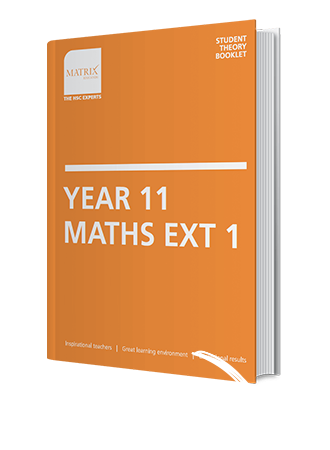# What our Year 11 Maths Extension 1 students will develop

### Gain an in-depth understanding

Don’t just know the theory but know why and how. Gain a thorough knowledge of all syllabus dot points and outcomes for each module prescribed by the NSW Board of Studies.

### Learn to solve unfamiliar questions

Be equipped with the skills to solve new, unfamiliar and challenging Maths Ext 1 questions. All Matrix students have access to the resources online to practice and further develop their mathematical learning.

#### No more guesswork

Look forward to showing off your Maths skills in school and at Matrix. With the best tools and support on your side there’s nothing to fear!

## What our Year 11 Maths Extension 1 students receive

Comprehensive Theory Book

Exam-Style Workbook

Weekly Mathematics Quiz

One-to-One Tutorials (Workshops)

End of Term Topic Test

Exclusive Online ResourcesYear 11 Maths Extension 1 Term Course Program (New Syllabus)

#### Topics

Functions and Relations

Non-Linear Functions

Absolute Values and Inequalities

Polynomials

#### Topics

Trigonometry and Inverse Trigonometry

Introductory Calculus

#### Topics

Applications of Differentiation

Exponentials and Logarithms

Motion in a Straight Line

Rates of Change

Graphical Transformation

#### Topics

Parametric Forms

Probability and Statistics

Permutations and Combinations

Binomial Expansions

## Year 11 Maths Ext 1 Term Course Details (New Syllabus)

9 weekly lesson in the school term

#### Oct - Dec

Functions & Relations, Non-Linear Functions, Absolute Values & Inequalities and Polynomials

Lesson 1: Functions and Relations

Lesson 2: Linear Functions

Lesson 4: Polynomials 1

Lesson 5: Polynomials 2

Lesson 6: Other Non-Linear Relations

Lesson 7: Absolute Values

Lesson 8: Inverse Functions

Lesson 9: Review and in-class Topic Test

#### Feb - Apr

Trigonometry & Inverse Trigonometry and Introductory Calculus

Lesson 1: Trigonometry 1

Lesson 2: Trigonometry 2

Lesson 3: Trigonometry 3

Lesson 4: Trigonometry 4

Lesson 5: Inverse Trigonometry

Lesson 6: Introductory Calculus 1

Lesson 7:Introductory Calculus 2

Lesson 8:Introductory Calculus 3

Lesson 9: Review and in-class Topic Test

#### Apr - Jun

Graphical Relationships, Applications of Differentiation and Exponential & Logarithms

Lesson 1: Exponentials and Logarithms

Lesson 2: Derivatives of Exponentials and Logarithms

Lesson 3: Calculus and Curve Sketching 1

Lesson 4: Calculus and Curve Sketching 2

Lesson 5: Further Applications of Calculus 1

Lesson 6: Further Applications of Calculus 2

Lesson 7: Graphical Relationships 1

Lesson 8: Graphical Relationships 2

Lesson 9: Review and in-class Topic Test

#### Jul - Sep

Parametric Forms, Probability & Statistics and Combinatorics

Lesson 1: Parametric Representation

Lesson 2: Probability 1

Lesson 3: Probability 2

Lesson 4: Statistics 1

Lesson 5: Statistics 2

Lesson 6: Combinatorics 1

Lesson 7: Combinatorics 2

Lesson 8: Binomial Expansion

Lesson 9: Review and in-class Topic Test

## 3 Hour Lesson Breakdown

Surprise yourself with how much you can learn in 3 hours

 30 min Introduction Revision, Quiz and OverviewTeachers revise previous lesson and answer homework questions before conducting an in-class quiz. Teachers revise previous lesson and answer homework questions before conducting an in-class quiz. 65 min Theory Lesson Part 1 Gain in-depth knowledge and understandingTeachers explain key mathematics concepts which are reinforced by completing exam-style questions. Teachers explain key mathematics concepts which are reinforced by completing exam-style questions. 10 min Break 65 min Theory Lesson Part 2 Gain in-depth knowledge and understandingTeachers explain key mathematics concepts which are reinforced by completing exam-style questions. Teachers explain key mathematics concepts which are reinforced by completing exam-style questions. 10 min Review Q&AStudents ask questions to clarify understanding. Students ask questions to clarify understanding.

## Year 11 Maths Extension 1 Timetable

9 weekly lessons for comprehensive knowledge and understanding.

per Hour

x

per Lesson

x

per Term

=

## \$1215

per Term (inc. GST)

What you'll get

• #### Additional online resources including practice papers and solutions

Love us or walk away!

All courses come with a First Lesson Money Back Guarantee.

## How is Matrix different?

MatrixOthers##### Teachers

Matrix

Vast classroom teaching experience, HSC and/or university teaching experience with an ability to explain concepts clearly

Others

Have little or no actual teaching experience, cannot explain difficult concepts in a manner that students can understand##### Program

Matrix

Teaching program that covers the NSW board of studies syllabus. Structured learning system that allows students to develop sound study habits every week

Others

Most private tutors and other tuition centres do not follow a set structure; rather they have an ad-hoc approach without considering timing and outcomes##### Resources

Matrix

A full set of resources written by academics and education researchers. Online access to Supplementary exam papers / texts with top responses.

Others

Only address content that students raise, use generic textbooks.##### Assessments

Matrix

Short quizzes and topic tests held under strict exam conditions to ensure students have a solid understanding of the subject

Others

No exam style quizzes and test that assess the student’s weaknesses, hence not being able to identifying their learning needs##### Reporting

Matrix

Weekly tracking of Grades, Quiz and Topic Test results so student and parents can monitor their progress.

Others

No measured tracking process so they cannot tell if the student is struggling or improving.##### Learning Management System

Matrix

Share information, academic resources and advice with classmates from over 220 schools.

Others

Do not have the library of resources or past exams that can help students excel##### One-to-One Workshops

Matrix

Free workshops to target individual learning needs

Others

No additional support outside the lesson.